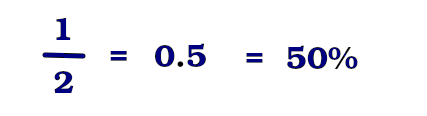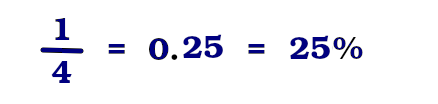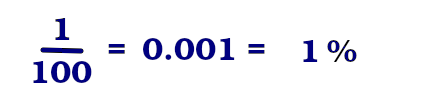# How to calculate percentages## What are percentages?#

Every person in their daily life comes across phrases or conversations involving the percentage concept. And we usually do understand this concept without any difficulties.

• 70% of the land was allocated for residential buildings
• Over the past six months we have increased revenue by 20%
• Interest on the deposit is 15% per annum
• The human body consists of 60-85% water

And so on..

Nevertheless, some people don't get this concept fully and face difficulties when they have to apply percentages in their calculations.
In particular I want to answer the following questions:

• What are percentages?
• How to calculate them?
• How should one apply them in practice?

## Percentages come to the rescue#

Percentages allow us to talk about quantity, size, volume, etc. without having to give the exact numerical value. It is much easier to provide relative values than it is to deal with exact (often large) figures.

A very simple example of a relative measure is half of something.

• half a glass,
• half an apple,
• half a budget,
• half a salary, etc.

As you may notice, nothing was said about the exact volume of a glass, the size of an apple or the actual salary, but only about relative size of those things in relation to them as a whole. Note that half of something in percentages is equal to 50%. In fact, half and 50% are synonyms.

Another similar example is a quarter or one fourth of something. As in the case with halves, a quarter corresponds to a certain percentage, i.e. 25%.

Let’s summarize:
Percentage gives us an idea of the quantity or size of something without specifying the exact value (It’s worth mentioning that precise value can still be obtained from percentage, but we will talk about this a bit later). It allows us to describe quantitative values ​​in relative units, i.e. in percentages.

### Percentage definition#

Percentage (from novolat. per centum, i.e. 'per hundred; hundredth') indicates the proportion of something in relation to the whole taken as a hundred.

As you can see, this statement include two main components:

• The whole means the entire volume or sum of something taken as a hundred. It can be \$1 million or 3 560 870 electoral votes of the state or 700 hectares of land etc.
We always take this whole as a hundred whatever it will be.
• The part (or portion) of this whole.We can also notice two more things here:

1. the whole is always 100%
2. one hundredth of that whole is always 1%

For example, if we are talking

• about a glass of water, then the volume of the whole glass is 100%
• about an apple, then 100% will be the size of the whole apple (diameter or weight of the apple)
• about a budget, then the entire budget (at a given time) is taken as 100%
• about a salary, then the entire salary (at a given moment) is taken as 100%

### How can we express a portion of something in percentages?#

Let's take a look at fractions since percentages and fractions are related concepts. Moreover, the percentage can always be represented as a fraction or as a decimal number and vice versa.

Fractions are expressed in fractional numbers, for example:

Half - is one divided by two ½ (in decimal form 0.5)Quarter - is one divided by four. ¼ (in decimal form 0.25)One percent - is one divided by hundred or one hundredth of something, 1% is written as 1/100 in a form of a fraction while it is 0.01 in decimal form## What can we do with percentages ?#

Besides some sophisticated math stuff, percentages can help us with these three main questions:

Let’s now address each of these points on the example of spending a week in the store.

During the week \$800 were spent on various needs and \$160 of those expenses were on groceries.### Finding percentage of a part#

When we know a part and a whole number and want to know the percentage of that part in relation to the whole. In our case the question is: What is the percentage of the expenses?

To calculate percentage of a number, we need to proceed in the following way:

step 1: Find the proportion of a part to a whole number by dividing the value of a part by the value of a whole number.

160/800 = 1/5 = 0.2

At this step we get a fraction 1/5, which in decimal form is written as 0.2

step 2: Convert obtained decimal number to a percentage by multiplying it by 100

1/5 * 100 = 20%

At this step we convert the fraction to the actual percent. Thus we spend on groceries 20%.

Full solution: 160 / 800 * 100 = 20%

In Bricks calculator we can get the same result by using the following built-in formula:

part number of whole number to %

160 of 800 to % |20%

### Finding a part knowing its percent and a whole number#

When we know the whole number and the percentage of the part and want to know what number represents that part.

Let’s suppose we know that we have spent 20% on groceries and we also know the overall expenses were \$800. In our case the question is: How much did we spend exactly on groceries?

To calculate this we need to take the following steps:

step 1: Convert the percentage to the proportion by dividing percentage value by 100

20%/100 = 1/5 = 0.2

step 2: Multiply obtained decimal number by the overall sum

0.2 * 800 = 160

Full solution: 20% / 100 * \$800 = \$160

In Bricks calculator we can get the same result by using the following built-in formula:

percentage of whole number

20% of 800 |160

### Finding a whole number knowing the percentage of a part#

Let’s say we know that we have spent 20% on groceries and we also know its exact value is \$160. In our case the question is 'How much did we spend for the given week on the whole?'

To calculate the whole sum, again we take two steps:

step 1: Convert the percentage to the proportion by dividing 100 by percentage value

100 / 20% = 5

At this step we get a number that indicates how many times the value of the part is given in the whole number

step 2: Multiply obtained number by the value of the part

5 * \$160 = 800

Full solution: 100 / 20% * \$160 = \$800

In Bricks calculator we can get the same result by using the following built-in formula:

part number of percentage of

160 is 20% of | 800